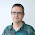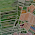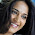## Monday, December 10, 2012

### Raster calculations with GDAL and numpy: calculating GFS wid speed

Performing raster calculations is a frequent need. GDAL python bindings come with a utility program,  gdal_calc.py, that does that, but many times is useful to code them.
In this example, we will be able to calculate the wind speed field from the GFS model, from the given u and v speed.
The calculated wind field. America is easily seen at the right side of the image

### The data

In this example, we will use the GFS global model data, which is available at their web site. Just navigate to a date that pleases you and download a .grb file.
Files are in grib format, with a little problem when trying to view them in QGis: Coordinates are from 0 to 360º in longitude, while the usual thing is to put them from -180º to 180º. Everything will work without changing this, but you can do it after taking a look to this post.

Inside the grib file, there are many bands (hundreds). You can see their definition using the gdal command gdalifo, or the file with the extension *inv at the same place you downloaded the grib file. There are many wind fields, that correspond to different altitudes, all them indicated as u (east) and v components (north). Just take note of the band number of both at the desired level.

### NumPy

The best of using the python gdal bindings, compared from those of the other programs, is that converting a raster file to a NumPy matrix is now very easy.
NumPy is a python extension that allows to perform matrix calculations in a really easy and efficient way. It should be installed in your computer if GDAL python is installed.
Once you have two matrices A and B in NumPy, adding element by element to a C matrix would be as easy as:
C = A + B
and multiplying:
C = A * B
Note that is not a matrix multiplication (more info here), but this is what we usually need when working with raster images.

### The code

The wind speed is the modulus of the two components, so
spd = sqrt(u^2+v^2)
The "traditional way" to calculate it would be opening two bands and loop all the elements to create the new band. Instead, this can be done:
from osgeo import gdal
from osgeo.gdalnumeric import *
from osgeo.gdalconst import *

fileName = "gfs.tiff"
bandNum1 = 199
bandNum2 = 200

outFile = "out.tiff"

#Open the dataset
ds1 = gdal.Open(fileName, GA_ReadOnly )
band1 = ds1.GetRasterBand(bandNum1)
band2 = ds1.GetRasterBand(bandNum2)

#Read the data into numpy arrays

#The actual calculation
dataOut = numpy.sqrt(data1*data1+data2*data2)

#Write the out file
driver = gdal.GetDriverByName("GTiff")
dsOut = driver.Create("out.tiff", ds1.RasterXSize, ds1.RasterYSize, 1, band1.DataType)
CopyDatasetInfo(ds1,dsOut)
bandOut=dsOut.GetRasterBand(1)
BandWriteArray(bandOut, dataOut)

#Close the datasets
band1 = None
band2 = None
ds1 = None
bandOut = None
dsOut = None


Note that:
1. gdalnumeric is used, because it's the easiest way to dump a raster into a NumPy matrix using BandReadAsArray and the inverse function with BandWriteArray.
2. In this case, we are reading the bands 199 and 200, but you should check which bands are the ones to read in the file you download.
3. CopyDatasetInfo will copy all the input file metadata into the output file. This includes the geotransform, the projection, the GCPs (there aren't in this case) and the other metadata.
4. Don't forget to set the bands and datasets to None at the end to free them. In longer scripts this may be critical.

### gdal_calc.py

If you want to use the command line function to do the same, you can try with:
gdal_calc.py -A gfs.tiff --A_band 199 -B gfs.tiff --B_band 200 --outfile out.tiff --calc="sqrt(A*A+B*B)"
It gives the same result.

1.Hi,

thanks for this post, very clear.

I am a math teacher and, to introduce the concepts of vector field / ordinary differential equations,
I'd like to plot a wind field over our french Brittany region. Here are the coordinates using the basemap module
notations :

urcrnrlon=-2.6, urcrnrlat=49.0,
llcrnrlon=-5.34, llcrnrlat=47

Yet I am a newbie in the geosciences area. So I think (tell me please if I am wrong) the workflow is the following :

1. Get a grb file as you said,
2. Extract from this file the geographic zone I am interested in (Brittany coasts)
3. Generate the raster for the wind field (corresponding the gfs.tiff file you used).
4. Make computations as you did in your script (essentially compute the modulus of the vector field)

I have no idea with 2 and 3 ... (since 1 and 4 are trivial thanks to your post !)

Last question : how does the gdalifo command works ?

Thanks a lot !

Pr. Yassine Patel
yaspat.github.io/blog

1.Hi Yassine,

I think that using the Basemap library will make your life easier. This example will plot you the results in the frame defined when you create the Basemap instance: https://basemaptutorial.readthedocs.org/en/latest/plotting_data.html#contourf
Of course, you have to change the data array to whatever you need (the module, in your case).
I like using basemap, since you can show the wind direction too:
or, even prettier:

I'm happy to know that the blog posts can be used to teach!

2.Hi,
can we create mosaic dataset in gdal or numpy?
thanks

3.if gdal cannot read raster as array, how could we use raster calculator using gdal?

1.Which data format are you using? If GDAL can't read it through python, it won't read it neither with the command line utilities.
Maybe you can transform your file into GeoTIFF or any other available format. Usually, ENVI format is an easy one.

4.Hello Roger,

I am using a SAR CEOS data file which comes with leader file, volume directory file and null volume directory file.

I am opening a data file using gdal ReadasArray and doing operations on that 2d array,after that I want to save this 2d Array as an ENVI binary file.

Kindly guide how to do this.

5.I like your blog, I read this blog please update more content on python, further check it once at python online training